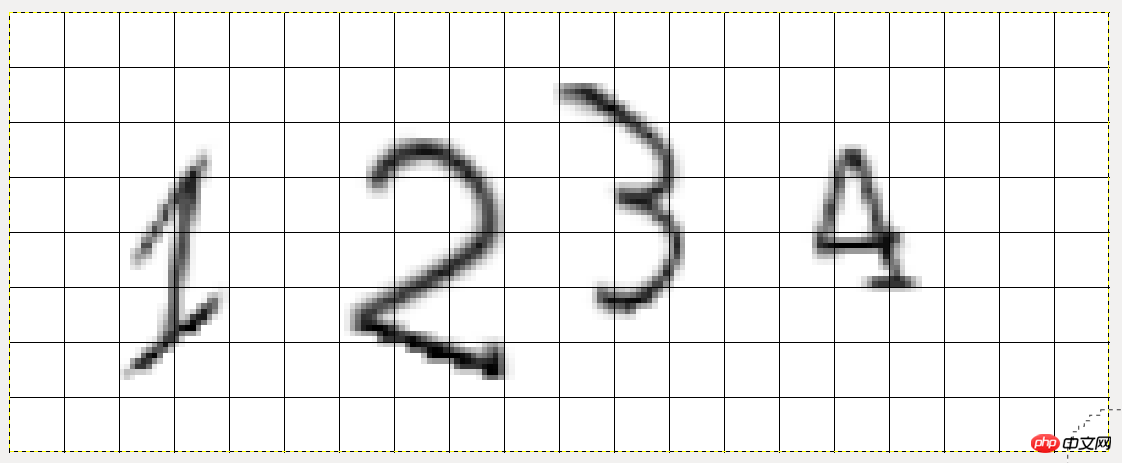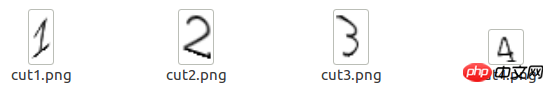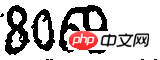# python验证码识别教程之利用投影法、连通域法分割图片```from PIL import Image
p = Image.open("1.png")
# 注意位置顺序为左、上、右、下
cuts = [(20,20,40,70),(60,20,90,70),(100,10,130,60),(140,20,170,50)]
for i,n in enumerate(cuts,1):
temp = p.crop(n) # 调用crop函数进行切割
temp.save("cut%s.png" % i)``````def vertical(img):
"""传入二值化后的图片进行垂直投影"""
w,h = img.size
ver_list = []
# 开始投影
for x in range(w):
black = 0
for y in range(h):
if pixdata[x,y] == 0:
black += 1
ver_list.append(black)
# 判断边界
l,r = 0,0
flag = False
cuts = []
for i,count in enumerate(ver_list):
# 阈值这里为0
if flag is False and count > 0:
l = i
flag = True
if flag and count == 0:
r = i-1
flag = False
cuts.append((l,r))
return cuts

p = Image.open('1.png')
b_img = binarizing(p,200)
v = vertical(b_img)```

`[(21, 37), (62, 89), (100, 122), (146, 164)]``[(5, 27), (33, 53), (59, 108)]`

`[(5, 27), (33, 53), (60, 79), (83, 108)]`

• 将二值化后的图片进行从左到右、从上到下的遍历，如果遇到黑色像素并且这个像素没有没访问过，就将这个像素入栈并标记为已经访问。

• 如果栈不为空，则继续探测周围8个像素，并执行第2步；如果栈空，则代表探测完了一个字符块。

• 探测结束，这样就确定了若干字符。

```import queue

def cfs(img):
"""传入二值化后的图片进行连通域分割"""
w,h = img.size
visited = set()
q = queue.Queue()
offset = [(-1,-1),(0,-1),(1,-1),(-1,0),(1,0),(-1,1),(0,1),(1,1)]
cuts = []
for x in range(w):
for y in range(h):
x_axis = []
#y_axis = []
if pixdata[x,y] == 0 and (x,y) not in visited:
q.put((x,y))
while not q.empty():
x_p,y_p = q.get()
for x_offset,y_offset in offset:
x_c,y_c = x_p+x_offset,y_p+y_offset
if (x_c,y_c) in visited:
continue
try:
if pixdata[x_c,y_c] == 0:
q.put((x_c,y_c))
x_axis.append(x_c)
#y_axis.append(y_c)
except:
pass
if x_axis:
min_x,max_x = min(x_axis),max(x_axis)
if max_x - min_x > 3:
# 宽度小于3的认为是噪点，根据需要修改
cuts.append((min_x,max_x))
return cuts```